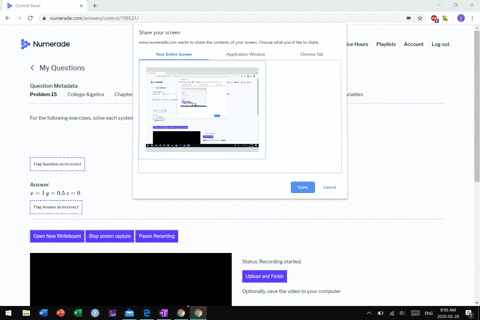Sign up for our free STEM online summer camps starting June 1st!View Summer Courses### For the following exercises, solve each system by…

02:17
MB

Need more help? Fill out this quick form to get professional live tutoring.

Get live tutoring
Problem 14

For the following exercises, solve each system by substitution.
\begin{aligned} 4 x-3 y+5 z &=31 \\-x+2 y+4 z &=20 \\ x+5 y-2 z &=-29 \end{aligned}

$x=-2$
$y=-3$
$z=6$

## Discussion

You must be signed in to discuss.

## Video Transcript

All right, guys, to solve this system of equations, you see, substitution, um, got really carefully. You don't make silly mistakes. I'm doing this whole problem a second time for you right here, because I forgot a negative sign. Um, about 4/5 of the way through the problem. So when you're doing them, just be really careful that you're not making a stealing state somewhere along very hard not to, but it is what it is. So solving this by by substitution, basically, what we have to do. Is it an equation? One of these equations solve it or one of the variables. Um, I'm electing to do this one because I see the exes by itself already. So it's easy to solve for so I get eggs equals negative five. Why? Plus two z, uh, minus 20. All right, So what I'm gonna do then is and I'm gonna take what this last equation says X is equal to that. Might substitute that in for my two exits up here. All right, so the first equation turns into, uh, four times x of war, and I'm gonna put this mess in there, so maybe get five. Why? Plus to the one is 29. Okay. Um, equals Oh, sorry. Plus minus three. Why? Plus five c equals 31. Okay, um, so let's clean this up before him around to the other equation. So this is negative. We won. And then plus he z four times to people five didn't get do four times. Native 29. Um, Thio 1 16 B minus 1 16 Then my mystery. Why us See? Equals 31 um, combined, Like terms. So that negative 23. Why? And then ese for spies e equals. And then I had 1 16 31 which is 1 47 All right, so that's the first equation. So then next thing I knew, I needed something to my ex into this equation here. So negative. Then we'll have to put in my expression for X negative five z plus by why plus two z minus 29. Okay. And then I've still got the plus two wine can plus four z equals 20. All right. Need district distribute this negative. So I get 51 minus two z plus 29 plus two. Uh, plus for Z. Who's 20? I looked like terms. Five wife was too wise. You seven. Why? A negative to Z or Z is a to Z and that's been the equal. Subtract with nine both sides not and need to solve this for my two variables else. All this Z hey Z is kind of people Negative nine minus seven. Why or two? What? And so this expression now this expression goes in for my c here. That's what I would do that. So make it one three. What? Plus 13 times my new expression for Izzy, which is negative. Nine minus 71 over too equals 1 47 All right, um, you see to those into 13 6 and 1/2 times. So I now have to distribute mind 6.5. This is down that we three. Why? So? 6.5 times? Maybe not? Yes, a negative 58.5 and then 6.5 times maybe seven mines. So is 45.5. Why C minus five y. Then we'll get our wise together into the 68. Negative. 68.5. Why then at 58. 15 five What? And they divide that by negative 65. He says why equals negative three s? That's where I made my mistake the last time I didn't put in the making good thing over again. All right, so now I'm ready to go over here looking my wife. And so I get Z is equal to mine. Minus seven times nated Over too. So negative nine from plus 21. I'm about to six. So Z will six and then finally x So actually equal. And it is fire turns. What? And then plus two plants, my Z, which is six minus 29. So, huh, this'd deposit 15 was 12 is 27 minus 29. So x equals negative, too. So my order triple that solves this whole thing is since negative to deepens the why I'm busy, all right?